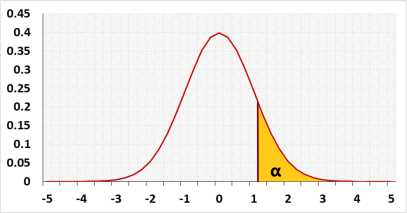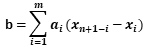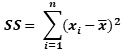Shapiro-Wilk Test Calculator

The maximum sample size is 5000

Target: Check if the normal distribution model fits the observations
The tool combine the following methods: Formal normality test: Shapiro-Wilk test results, this is one of the most powerfull normality tests. Graphical methods: QQ-Plot chart and Histogram

Hypotheses

 H0: Normal Distribution H1: Other Distribution

Test statisticDistributionTest calculation

Statistic Data

 Name: The name of the group you sampled from α: Significant level (0-1), maximum chance allowed rejecting H0 while H0 is correct (Type1 Error) Outliers: IncludedExcluded It is not recommended to exclude outliers unless you know the reasons

Enter sample data

When entering data, press Enter after each value or copy data from Excel, Google sheets or any tool that separate data with Tab. When copy the data, choose one block includes the header (or only data), and paste below.

It is okay to leave empty cells, empty cells or non numeric cells won't be counted

Results

 n: sample size x: sample average median: sample median S: sample standard deviation SS: Sum of Squares b: b statistic skewness: Excess kurtosis: p-value: the probability the data was sampled from a normal distribution Outliers observation point that is distant from other observations
validation message